﻿ Synchronization of Two Identical Wang Chaotic Systems via Active Sliding Mode Control

### Synchronization of Two Identical Wang Chaotic Systems via Active Sliding Mode Control

Journal of Automation and Control

## Synchronization of Two Identical Wang Chaotic Systems via Active Sliding Mode Control

Fayiz Abu KhadraMechanical Engineering Department, King Abdulaziz University, Rabigh, Saudi Arabia

### Abstract

This paper investigates the design of active sliding mode controllers to synchronize two identical Wang chaotic systems. The switching function matrix is determined based on linear quadratic minimization process. The stability of the controllers is established using the Lyapunov stability theory. Numerical results are shown to demonstrate the effectiveness of the proposed control method.

• Fayiz Abu Khadra. Synchronization of Two Identical Wang Chaotic Systems via Active Sliding Mode Control. Journal of Automation and Control. Vol. 5, No. 1, 2017, pp 1-6. http://pubs.sciepub.com/automation/5/1/1
• Khadra, Fayiz Abu. "Synchronization of Two Identical Wang Chaotic Systems via Active Sliding Mode Control." Journal of Automation and Control 5.1 (2017): 1-6.
• Khadra, F. A. (2017). Synchronization of Two Identical Wang Chaotic Systems via Active Sliding Mode Control. Journal of Automation and Control, 5(1), 1-6.
• Khadra, Fayiz Abu. "Synchronization of Two Identical Wang Chaotic Systems via Active Sliding Mode Control." Journal of Automation and Control 5, no. 1 (2017): 1-6.

 Import into BibTeX Import into EndNote Import into RefMan Import into RefWorks

12
Prev Next

### 1. Introduction

A Chaotic system is a dynamical system that is highly sensitive to initial conditions. The response of a chaotic system exhibits a number of specific characteristics: (i) A small change in the initial conditions leads to extraordinary differences in the system state; (ii) broad Fourier transform spectra (iii) and fractal properties of motion in phase space. Chaotic behavior can be found in many different systems, such as climate modeling . compressed beams , chemical systems , secure communications , etc.

Chaos synchronization is to make the response of two chaotic systems oscillate in a synchronized manner. Using a controller or more than one controller the states of the slave system are forced to follow the states of the master system. Since the pioneering work by Pecora and Carroll , chaos synchronization problem has been widely and intensively studied in the literature. In the last two decades, a number of control techniques have been successfully applied for chaos synchronization. Synchronizing chaotic systems using backstepping control has been utilized for several systems such as Lorenz system, Chua’s circuit and Duffing system in . The synchronization of the new hyperchaotic Liu system using backstepping techniques .The partial and full sinusoidal synchronizations of the controlled Duffing oscillator under specific function constraints was conducted in . A linear state error feedback control technique was utilized to synchronize two parametrically excited non-autonomous Duffing oscillators . A robust active controller with an integral high order sliding-mode and proportional form of the so-called control error was proposed . Robust indirect model reference fuzzy control scheme for control and synchronization of chaotic nonlinear systems subject to uncertainties and external disturbances was proposed in . A novel observer-based finite-time control method for the modified function projective synchronization of chaotic systems in the presence of uncertainties and external disturbances was presented in . In  A robust adaptive PID controller design motivated from the sliding mode control is proposed for a class of uncertain chaotic systems. Backstepping control method to derive new results for the adaptive controller and synchronizer design for the Arneodo chaotic system was used in  in  A generalized synchronization of coupled periodically driven duffing oscillators is investigated through Linear Matrix Inequality method. A control scheme based on a high order sliding-mode observer-identifier and a feedback state controller is proposed . In  the dislocated feedback control, enhancing feedback control and speed feedback control for controlling chaos in a unified chaotic system were used. An adaptive synchronization and parameters identification scheme was proposed in  for two coupled chaotic Newton-Leipnik systems. In  the synchronization of Nuclear spin generator system was investigated. In  a new scheme for constructing bidirectional nonlinear coupled chaotic systems which synchronize projectively was presented.

In this paper, the active control  and sliding mode control method ,  are used for the chaos synchronization of two identical Wang four-scroll chaotic systems. The basic idea of active control is the elimination of all the terms which cannot be written in term of the errors in the error dynamics. The design of a sliding mode controller consists of two steps. The first step is the selection of a switching surface for the desired dynamics and the second step is to design a control law such that the system trajectory reaches the surface and stays in it forever. To design the sliding surface the minimization of the quadratic performance index is considered. The stability of error dynamics was checked based on the Lyapunov stability theory. Numerical simulation of synchronization system illustrates the effectiveness of the sliding mode control method.

This organization of this paper is as follows. In Section 2, the Wang chaotic system is described. In Section 3 the development of the sliding mode controllers is presented. In Section 4, the numerical results are presented. Section 5 concludes the paper.

### 2. Problem Statement and System Description

The problem discussed in this study concerns with master-slave synchronization of the new Wang four-scroll system chaotic system . A master Wang system is described as follows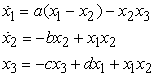(1)

where x1(t), x2(t), x3(3) are the states and a, b, c, d are positive, constant parameters of the system. The system (1) is chaotic when the parameters of the system are a=1, b=5.7, c=5 and d=0.06. Figure 1 depicts the four-scroll attractor of the Wang chaotic system (1).Download asVeiw figureFigures index
Figure 1. The four-scroll attractor of the Wang system

The slave system is described by the controlled Wang dynamics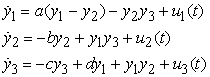(2)

where y1(t) , y2(t) , y3(t) are the states and u1 (t), u2 (t), u3 (t) are the controllers to be designed. We define the synchronization error signals as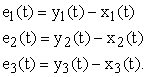(3)

Differentiating (3) with respect to time, and using (1) and (2), one obtains the error dynamics system given by: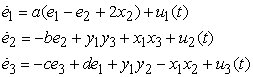(4)

The error dynamics can be written in matrix notation as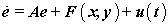(5)

whereSynchronization is to design an appropriate controllers vector u(t) so that for any initial conditions of the two systems the states of the slave system (2) asymptotically approaches the states of the Master system (1). Such that the resulting error vector satisfies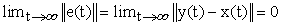(6)

wheredenote the Euclidean norm.

### 3. Active Sliding Mode Control Design

3.1. Active Control

According to the method of active control proposed in , a control input can be used to eliminate all terms that cannot be shown in the form of the error vector e(t). In this way, the control u(t) can be formulated as: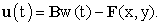(7)

Substituting (7) into (5) the error dynamics can be written as(8)

The error dynamics is now a linear time-invariant control system with single input w(t). As long as these feedbacks stabilize the system, the error vector e(t) converges to 0 as. This implies that the master system (1) and the slave system (2) are synchronized.

3.2. Sliding Mode Control

The design of a sliding mode controller consists of two steps: i) Design of a proper switching surface such that the closed-loop system behaves as desired (ii) Reach the switching surface in finite time and maintain it there (positive invariance).The sliding mode control law, as follows:. Thus, the original global chaos synchronization problem can be replaced by an equivalent problem of stabilizing the zero solution e = 0 of the system (8) by a suitable choice of the sliding mode control.

In the sliding mode control, we define the switching function to be :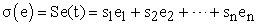(9)

whereis a constant row vector to be determined so that the product SB is a square nonsingular matrix (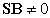) is a necessary condition. A necessary condition for the state trajectory to stay on the switching surface is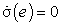is and. Therefore, when in sliding mode, the controlled system satisfies the following two conditions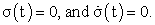(10)

Differentiating (9) with respect to time and substituting (8) yields(11)

Solving (11) for w(t), we obtain the equivalent control law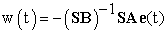(12)

w(t) is the control action required to maintain the state on the switching surface. The sliding mode motion is obtained by substituting (12) into the error dynamics (8) resulting in the closed-loop dynamics as: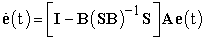(13)

where I is an identity matrix. The sliding motion depends on the matrix S.

3.3. Design the Sliding Surface via LQ Minimization.

It is well known that for the controllable system (8) there exists a nonsingular transformation, defined by(14)

Such that(15)

So that system (8) can be transformed into the following regular form:(16)

The switching function can be written in the new coordinate system as: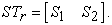(17)

During a sliding motion the following condition hold: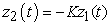(18)

where the matrix issubstituting (18) into the first equation of (16) yields(19)

Equation (19) is the reduced-order system which represents the motion equation in the sliding surface. If the pair (A, B) is controllable, then the pair (A11, A12) is controllable as well. Therefore, the design of sliding surfaces turn into choosing the matrix parameter K such that the sliding mode dynamics is stable. Finding the design matrix K is a classical state feedback problem with matrix K as a feedback gain and A12 as an input matrix. As a result various control methods based on state feedback are suitable to solve this problem. For example one can use the conventional eigenvalue allocation method or the linear-quadratic (LQ) approach. In this work, the design of the sliding surface subjected to minimization of the following quadratic performance index is considered .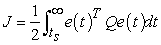(20)

where the matrix Q is a symmetric and positive matrix. Transforming the error dynamics into its regular form using the matrix Tr which can be obtained via QR factorization . The following can be written: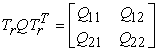(21)

The switching matrix can be calculated as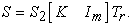(22)

The Matrix K is defined as: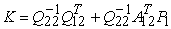(23)

P1 is a positive definite solution to the algebraic matrix Riccati equation(24)

Where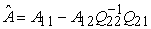and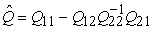.

3.3. Design of a Sliding Mode Controller

A straightforward method of sliding mode controller design is the so called reaching law approach. The reaching law is a differential equation which determines the dynamics of a switching function. From the different laws available the constant plus proportional rate reaching law is used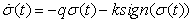(25)

where sign(⋅) denotes the sign function and given by:(26)

The gains q > 0, k > 0 are positive real numbers and determined such that the sliding condition is satisfied and sliding motion will occur. The selection of the parameters of the reaching law controls the dynamic quality of SMC system in the reaching mode.

Substituting (11) and (9) into (25) the control signal w(t) is obtained and can be expressed as follow(27)

Substituting (27) into (8) yields the closed loop error dynamics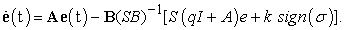(28)
3.4. Stability Analysis

In order to check that the error dynamics (28) is globally asymptotically stable, we choose the Lyapunov function defined by(29)

which is globally defined, positive definite function on Rn. Differentiating V(e) with respect to time, and substituting (28) and (9) into the differential results, we obtain(30)

which is always negative provided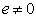, which means that the inequalityholds.

### 4. Numerical Results and Analysis

In this section, the numerical details of applying the sliding mode controllers derived in section 3, to chaos synchronization of two identical Wang systems are presented. The numerical simulations were carried out by employing a variable step Runge-Kutta Method. The initial values of the master system are set as, and the initial values of the slave system are taken as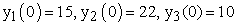.

The matrices A and B areThe LQ minimization matrix is.

The transformation matrices are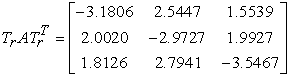After direct computation we have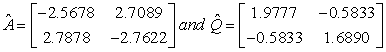and the matrix M is obtained as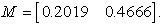Finally the matrix S isThe sliding mode variable is selected as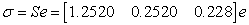Which make the sliding mode state equations asymptotically stable. The parameters of the sliding mode controller are selected as q=5 and k=0.2. Proper selection of the reaching Law’s parameters can reduce the chattering and the time required to reach the sliding surface. The controlled system (13) is globally asymptotically stable with the eigenvalues. From (27), we can obtain w(t) as(31)

Substituting the above equation in (7) the sliding mode controllers are obtained.

The complete synchronization of the states of the identical Wang system are shown in Figure 2. Figure 2a shows the complete synchronization of the states x1(t) and y1(t). Figure 2b shows the complete synchronization of the states x2(t) and y2(t). Figure 2c shows the complete synchronization of the states x3(t) and y3(t). It is seen that the states are synchronized in very short time after the activation of the controllers. Figure 3a, b, c display the time-history of the synchronization errors e1(t), e2(t), e3(t), respectively. It can be observed that the errors converge to zero rapidly. Figure 4 shows the evolution of the sliding surface (function with time). Figure 5 shows the control signals as function of time. From the figure presented it is evident that active sliding mode controllers are very efficient in the synchronization of the two identical Wang systems.Download asVeiw figureFigures index
Figure 2. The Time Responses of the States with Controllers Activated at t = 20 SecondDownload asVeiw figureFigures index
Figure 3. Synchronization Errors of the Wang System as Function of TimeDownload asVeiw figureFigures index
Figure 4. The time Response of the Sliding SurfaceDownload asVeiw figureFigures index
Figure 5. The Time Response of the Control Signals

### 5. Conclusions

In this paper, a sliding mode controller has been proposed for the global chaos synchronization of two chaotic Wang systems. The switching function matrix was determined based on linear quadratic minimization process. The stability of the controller was analyzed based on Lyapunov stability theory. Results of the simulations showed that the sliding mode control method is very effective and convenient to achieve global chaos synchronization of the chaotic Wang systems. The presented controller can be used to synchronize other chaotic systems.

### References

  D. T. Mihailović, G. Mimić, and I. Arsenić, “Climate Predictions: The Chaos and Complexity in Climate Models,” Adv. Meteorol., vol. 2014, pp. 1-14, 2014.In article  F. Battelli, M. Feckan, and M. Franca, “On the chaotic behavior of a compressed beam,” Dyn. PDE, vol. 4, no. 1, pp. 55-86, 2007.In article  I. R. Epstein and K. Showalter, “Nonlinear chemical dynamics: oscillations, patterns, and chaos,” J. Phys. Chem., vol. 100, no. 31, pp. 13132-13147, 1996.In article  M. Feki, “Anadaptive chaos synchronization scheme applied to secure communication,” Chaos Solitons Fractals, no. 18, pp. 141-148, 2003.In article  X. Tan, J. Zhang, and Y. Yang, “Synchronizing chaotic systems using backstepping design,” Chaos Solitons Fractals, vol. 16, no. 1, pp. 37-45, 2003.In article  A. N. Njah, “Tracking control and synchronization of the new hyperchaotic Liu system via backstepping techniques,” Nonlinear Dyn., vol. 61, no. 1-2, pp. 1-9, Jul. 2010.In article  F. Min and A. C. J. Luo, “Sinusoidal synchronization of a Duffing oscillator with a chaotic pendulum,” Phys. Lett. A, vol. 375, no. 34, pp. 3080-3089, Aug. 2011.In article  X. Wu, J. Cai, and M. Wang, “Global chaos synchronization of the parametrically excited Duffing oscillators by linear state error feedback control,” Chaos Solitons Fractals, vol. 36, no. 1, pp. 121-128, Apr. 2008.In article  R. Aguilar-López and R. Martı´nez-Guerra, “Control of chaotic oscillators via a class of model free active controller: Suppresion and synchronization,” Chaos Solitons Fractals, vol. 38, no. 2, pp. 531-540, Oct. 2008.In article  M. A. Khanesar, M. Teshnehlab, and O. Kaynak, “Control and synchronization of chaotic systems using a novel indirect model reference fuzzy controller,” Soft Comput., vol. 16, no. 7, pp. 1253-1265, Jul. 2012.In article  X.-T. Tran and H.-J. Kang, “A novel observer-based finite-time control method for modified function projective synchronization of uncertain chaotic (hyperchaotic) systems,” Nonlinear Dyn., vol. 80, no. 1-2, pp. 905-916, Apr. 2015.In article  W.-D. Chang and J.-J. Yan, “Adaptive robust PID controller design based on a sliding mode for uncertain chaotic systems,” Chaos Solitons Fractals, vol. 26, no. 1, pp. 167–175, Oct. 2005.In article  S. Vaidyanathan, “Adaptive Backstepping Controller and Synchronizer Design for Arneodo Chaotic System With Unknown Parameters,” Int. J. Comput. Sci. Inf. Technol., vol. 4, no. 6, pp. 143-157, Dec. 2012.In article  S. Xu and Y. Yang, “Generalized synchronization of coupled Duffing oscillators: an LMI based approach,” in Proceedings of the 2010 American Control Conference, 2010, pp. 1761-1766.In article  A. Rodríguez, J. De León, and L. Fridman, “Synchronization in reduced-order of chaotic systems via control approaches based on high-order sliding-mode observer,” Chaos Solitons Fractals, vol. 42, no. 5, pp. 3219-3233, Dec. 2009.In article  M. A. Khan, A. K. Mondal, and S. Poria, “Three control strategies for unified chaotic system,” Int. J. Appl. Mech. AndEngineering, vol. 16, no. 2, p. 597, 2011.In article  M. A. Khan, “Adaptive synchronization of two coupled Newton-Leipnik systems with uncertain parameter,” Int. J. Basic Appl. Sci., vol. 1, no. 4, pp. 439-447, 2012.In article  M. A. Khan and S. Poria, “Generalized Synchronization of Nuclear Spin Generator System and the Application in Secure Communication,” J. Dyn. Syst. Geom. Theor., vol. 10, no. 1, pp. 53-59, May 2012.In article  M. A. Khan and S. Poria, “Projective synchronization of chaotic systems with bidirectional nonlinear coupling,” Pramana, vol. 81, no. 3, pp. 395-406, Sep. 2013.In article  E.-W. Bai and K. E. Lonngren, “Synchronization of two Lorenz systems using active control,” Chaos Solitons Fractals, vol. 8, no. 1, pp. 51-58, 1997.In article  Y. Shtessel, C. Edwards, L. Fridman, and A. Levant, Sliding Mode Control and Observation. New York, NY: Springer New York, 2014.In article  C. Edwards and S. Spurgeon, Sliding mode control: theory and applications. CRC Press, 1998.In article  L. Wang, “3-scroll and 4-scroll chaotic attractors generated from a new 3-D quadratic autonomous system,” Nonlinear Dyn., vol. 56, no. 4, pp. 453-462, Jun. 2009.In article